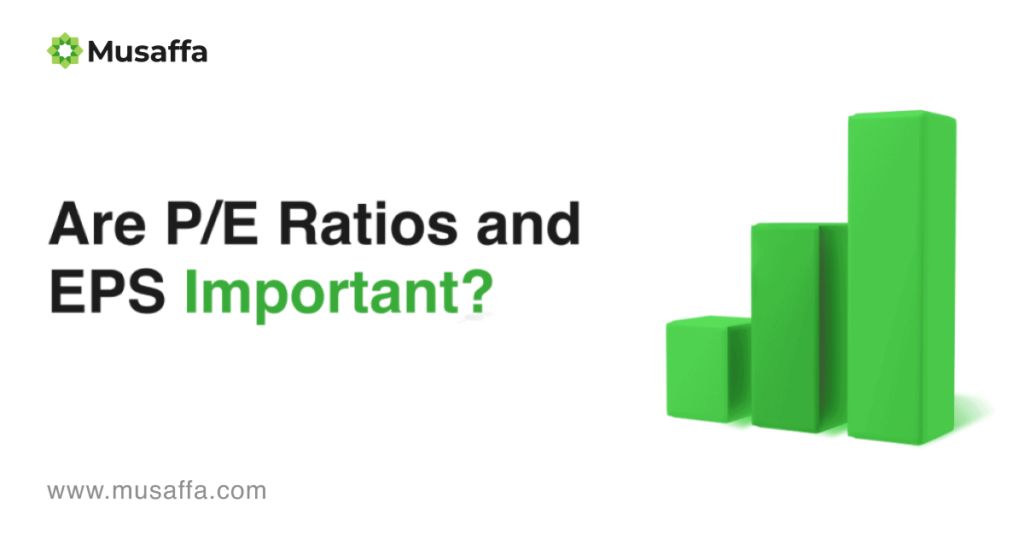# Are EPS and P/E Ratios Important?### Halal Investment Series Part 10

EPS and P/E ratios are very important to value investors when picking a stock. It helps investors determine if a stock is sold for overprice.

#### Earnings Per Share (EPS)

Earnings per share (EPS) measures how profitable a company is. You can find EPS by dividing the company’s profit by the outstanding shares. Looking at the EPS will tell you how profitable compared to the price.

It is also a key component in calculating the price-to-earnings (P/E) valuation ratio, where the E in P/E stands for earnings per share (EPS). An investor can determine the value of a stock by dividing its share price by its earnings per share. This metric shows how much the market is willing to pay for each dollar of earnings.

EPS is one of many indicators that to select stocks. Because ordinary shareholders do not directly access earnings, comparing EPS in absolute terms may be meaningless to investors. Instead, investors will compare EPS to the stock’s share price to determine the value of earnings and how investors feel about future growth.

EPS= (Net Income – Preferred Dividends) / End of period Shares Outstanding

#### Price-to-Earnings Ratio (P/E ratio)

EPS and P/E ratiosThe price-to-earnings ratio (P/E ratio) is a valuation ratio that compares a company’s current share price to its per-share earnings (EPS). The price-to-earnings ratio is also known as the earnings multiple or the price multiple.

Dividing the company’s share price by its earnings per share will give you the P/E ratio. For instance, if a company is trading at \$40 per share and earnings per share are \$2.50, the P/E ratio is 16.

P/E ratio = Stock Price Per Share / Earnings Per Share

A low P/E value can mean that the company is undervalued, and a high P/E value means it is overvalued. A lower P/E value can mean that you are getting that stock for a good price by comparing similar stocks.